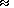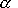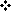Brief analysis of the state of the art   The principal feature of vibration processes is a great diversity of their forms with not so large number of the effecting parameters � such as the mass of system elements, stiffness of constraints, type of dissipative forces and so on. To outline the difference between the conventional approaches and results that we present in , , and , and that we have obtained by our new original non-matrix method, let us briefly analyse the state-of-the-art of this branch of the wave physics. From all the diversity we can pick a few basic conventional methods on which the entire spectrum of variations is built. To construct the mathematical model itself, two methods are used mainly: Lagrange method based on the energy balance and the method of forces balance at the elastic system elements. The first method advantage is a direct possibility to state the problem on the generalised co-ordinates for systems having many degrees of freedom. It makes this method profitable in the basic research of complex vibration processes. While the second method is more visual and simple when constructing the specific models of elastic systems, so it is used more often in applied developments. Both methods give similar results, so usually there are not considerable difficulties in their use at the stage of mathematical modelling. The difficulties arise when seeking the solutions. The conventional methods to seek the analytical solutions are based, as a rule, on one of three approaches to the solving the problem of a vibrant elastic system: �         With the matrix techniques direct use (see, e.g.,  � ); �         Using the techniques to solve the integral equations (see, e.g.,  � ); �         Using the indirect techniques based on the revelation of the regularities in specific modelling systems of differential equations (see, e.g., , , ). The solving of forced vibration problems is conventionally based on the studying of a homogeneous system of differential equations corresponding to free vibrations in an elastic model. The reason is the coincidence of the resonance frequencies for forced and free vibrations. So begin our brief analysis with such type of methods. Though the matrix method is thought conventionally analytical, in fact it is numerical, since the main vibration parameters can be obtained in general case only in numerical form. �The solving of these equations requires to find first the related determinants; in their written form and any considerable number of lines it is practically impossible or requires tremendous work� [6, p. 98]. �It goes without saying that a direct finding the determinant of 4n+4 elements (4n conjugation conditions plus 4 boundary conditions, � authors) would be impossible and helpless, as its root values might be calculated only numerically, not literally� [6, pp.278 � 282]. These computations are so laborious that going this way, one has to use all computers of his department at once to realise the parallel computing method developed just for this purpose. Furthermore, this demerit essentially limits the matrix techniques applicability to calculate the elastic systems having a finite number of elements. It also causes the development of such indirect techniques as described, e.g., in [6, p.161], [14, pp.95 � 99]. These techniques are grounded on some regularities revealed for the specific mathematical models, so they are essentially less applicable. None the less, for example, K. Magnus, when studying an infinite elastic lumped line, has obtained a solution not only for the subcritical band, which can be revealed by the matrix techniques, but also for the overcritical band, which cannot be revealed by the matrix techniques, because the natural frequencies are absent at this band. However, to find the natural frequencies by these techniques, one has to solve the power-type equation, for which one cannot conventionally yield analytically the solutions higher than of the fourth power. True, for today there is widely used a practice to extend the boundary conditions being true for distributed lines to those lumped. In particular, this procedure is used in [6, pp.149 � 150] and [11, pp.48 � 49], when studying a shaft having n fitted disks and a beam having n lumped masses relatively. None the less, as we proved in , when seeking the analytical solutions for a lumped line, one has to take into account the different boundary conditions for distributed and lumped lines. Almost nobody of the researches paid a due attention to the problem of the boundary conditions, but just this is the reason of many difficulties in the wave physics. Particularly, to write the modelling system of differential equations on generalised co-ordinates jointly with the initial and boundary conditions is an alphabet of the mathematical modelling. At the same time, applying this system of differential equations to any specific lumped model, we see that the system itself has already taken into account both initial and boundary conditions. It means that one reiterates the statement of problem. Should this reiteration be a duplication, there would be no problem. But as we showed in , e.g., for a finite line with unfixed ends, the boundary element vibration amplitude will be not maximal, as we used to think it for distributed lines. The vibrations of this element will delay by some phase depending on the action frequency of an external force and on the line discreteness. At the limit passing to a distributed line, this phase actually vanishes. But using the extreme condition to solve the problem with discrete elements, we automatically introduce to the solution an error increasing with the growing frequency. In case of more complicated boundary conditions, the solution errors will considerably increase, as generally the solution is not determined by the superposition of the direct and reflected waves, but as a rule is a result of multiple complex reflections from the boundaries. One cannot take it into account by a simple summing, the more that there will be particular features for a closed line, for a line having a heterogeneity transition they will be other, for a kinked elastic lumped line having inequal longitudinal and transversal stiffness coefficients they will be third, and so on. One more problem arises when using the matrix techniques. �The problem is solvable, if the forces and moments have been stated, but for a rotating shaft neither forces nor moments are stated, only their expressions through the shaft masses and moments of inertia and their deflections and inclinations at the fixation places� [6, p.167]. In this case, considering the system of equations composed for the boundaries of all homogeneous sections of a shaft, Krylov comes to the following conclusion: the resulting �expression will be so complicated that it will be possible to solve this equation only by way of sequential approximations� [6, p.173]. This demerit is inherent in the indirect techniques too. In most cases the solutions can be found only at the given amplitude and phase values � usually the boundary elements of a line. But in practical cases to give the external force and moments determining the initial vibration parameters is more important. And this problem is solvable in an exact analytical form only for some simple cases and under all above limitations. Regarding the conventional methods describing the infinite models, note that this area is even less clear. The solutions are mostly limited by finding the phase delay along an infinite elastic line and by the dispersion characteristics (see, e.g., [14, p.169], [15, pp.106 � 107]). At the same time Pain writes: �The most ultrasonic frequency produced at present is about 10 times less than that critical. In the band from 5.1012 to 1.1013 Hz one can expect many interesting experimental results� [14, p.169]. Actually, the results presented in  show that in infinite elastic systems both forced and free vibrations are possible. Note that they can exist not only at the subcritical band (lower than critical vibration frequency), as it takes place in finite lines, but also at the overcritical band, when all the vibration energy localises in a small vicinity of the external force application point, not distributed along an elastic line. In this case the energy accumulated in an infinite line can be finite. These conclusions are especially important in the view that many physical processes, e.g., in crystals, can be modelled just by an ideal infinite model. Moreover, this modelling is valid also for finite elastic lines loaded on the wave impedance. When using the method to solve the integral equations, the results are the same. �If an integral equation of the vibrant system with a continually-discrete mass distribution was constructed, then, using Fredholm method, one can find the eigenvalues. Actually, to find the eigenvalues, one needs to construct prematurely Green functions and to determine the complicated coefficients Cn, especially when finding the high-order eigenvalues. Note also that there is a whole class of the boundary problems for which one cannot construct so named generalised Green function� [11, p.38]. Using this method, Kukhta and Kravtchenko have reduced a specific problem on forced vibrations to the numerical modelling. �To find the roots (frequencies of the considered problem), their lower boundary is estimated, and then, combining the chord technique and choise technique conveniently realisable on computer in case of both simple and multiple roots, a necessary quantity of frequencies in an order of their increase is determined� [11, p.47]. Just as in matrix techniques, in this technique all above demerits are inherent, as it �works� only at the known eigenvalues which one must determine numerically. But not only demerits join these techniques. In essence, they are variations of the same approach. As a result, they both are able only predict in an analytical form that �if the damping is small, each amplitude will have s resonance peaks at s frequenciesca (= 1, 2, �, s). These maximums turn into infinity, if the energy dissipation was absent. As a counterpoise, the exact analytical solutions obtained by the original non-matrix method in ,  and , show not one but three vibration regimes: periodical (<0), critical (=0) and aperiodical (>0). Unfortunately, these two last regimes are not considered, nor taken into account both in practical investigations (see, e.g., , ) and in basic research (see, e.g., , ). �The solving of the boundary problems of vibration theory is reduced, in essence, to finding the eigenvalues connected with the natural frequencies or other parameters of the studied system and to finding the eigenfunctions (vibration forms). If the eigenvalues and eigenfunctions have been found, then one can think the boundary problem solved� For today a large number of approximation techniques to find eigenvalues has been developed, but they all are quite laborious, allow to find only the first eigenvalues, and the main, do not unite in the studying the systems having discrete and continuos mass distribution. So, as many authors correctly think, to find the eigenvalues is up to now one of the most important and at the same time laborious problems. So many researchers, in that number Kellatz, Guld, Wilkinson in their recent basic monographs devoted to the problem of eigenvalues, go on paying their attention to these problems. As long ago as Euler and Lagrange considered in their works, how to establish the mathematical unity and synthesis of 1D discrete and continuos boundary problems. But for the time being the establishment of an analogy between such boundary problems goes on in the works by, e.g., Crain, Atkinson and others� [11, pp.3 � 4]. A special place is given at present to asymptotic techniques, qualitative techniques, differential techniques etc. Although they are approximate, for today they are the only enabling to obtain solutions for the models with resonance subsystems, nonlinear models and others. �The classical vibration theory is based on the solution of differential equations and on joining the solutions for different parts of the system, basing on the continuity conditions. Any negligible change of the system form causes the necessity to compute all anew. But out of any connection with the greatly laborious computations, note that the high accuracy of the classical theory is illusory. Materials never are absolutely homogeneous or isotropic, and natural frequencies and vibration distributions usually considerably differ from those which theory gives, especially at high frequencies� [17, p.317]. At the same time, �multifrequent resonance systems are interesting by their applications to analytical and celestial mechanics, in Hamiltonian dynamics, in theoretical and mathematical physics� [18, 173]. In particular, the problem of discrete-continual elastic system vibration , of long molecular chains vibrations [17, p.317], of molecules vibration levels , of crystal lattice oscillations , , , problems in molecular acoustics , in quantum systems statistical mechanics , control problems  etc., etc. From the multitudinous approaches to the solving these problems, one can emphasise �such widely known vibration theory techniques as the perturbation theory, averaging technique, analytical techniques to separate slow and fast motions and so on� [25, p.45]. Each of these techniques has an extensive basis of sources. Tong Kin [26, p.45] devotes his investigation to pure matrix techniques; Kukhta and others  � to the solving by finding the recurrent relations; Atkinson  � to differential techniques; Palis and de Melo  � to geometric techniques; Reiscig and others  � to the qualitative theory. A good survey of solutions obtained by asymptotic techniques Mitropolsky and Homa  and Cherepennikov  gave. The techniques based on the perturbation theory are stated well by Giacagrilia  and Dymentberg . The approaches based on the elastic model presentation by mechanical resonance circuits are described quite completely by Skudrzyk . Despite the wide scope of approaches to the studied problem, all these techniques are qualitative, approximative or numerical. �The presence of irregular bounds in the most of practical problems disables us to construct an analytical solution of differential equations, and numerical techniques became the only possible means to obtain quite accurate and detailed results� [34, p.12]. �Even for the simplest case of hydrogen molecule H2 the exact quantum-mechanical calculation of the constant quasi-elastic force is a laborious mathematical problem, and for more complex cases the force constants calculation with the help of sequential quantum-mechanical techniques is practically unrealisable at all� [20, p.12]. �Another difficulty connected with the collective motions technique is that it does not allow to determine the collective motion nature, proceeding from the form of Hamiltonian. We must guess the proper collective variables and then check, does the Hamiltonian divide into the collective and interior parts� [24, p.120]. Enough complete analysis of the problems arising in the conventional approaches to the studying the multiresonance systems is given by Giacagrilia , Reiscig  and Cherepennikov . In particular, �an old problem retains open. Up to now no available �modern� techniques enable us to compute the real frequencies of a nonlinear system. For applications this problem retains unsolved, since in the approximations by series, converging or only formal, only finite and, generally speaking, small number of terms can be calculated. We cannot yet find a way to express the general term and the sum of these series� [32, p.305]. Furthermore, �to provide the series converging, sometimes we must presume that the parameters of differential equations determining the power of nonlinearity have quite small module. By this reason the indirect technique is often applicable only in a narrow boundary area of the nonlinear mechanics. Another demerit of these techniques is that they enable one to obtain quite accurate information about the separate solutions, but give no idea concerning the structure of the family of solutions as a whole� [29, p.12]. Giacagrilia confirms it: �Another problem of a great interest is the item of better understanding the solution �far, near and at the resonance conditions�. When we will really have a process of capture into the resonance, and which definition of the resonance is preferable?� [32, p.309]. �Exact analytical techniques are preferable in the analysis, however obtaining the analytical formulas even for comparatively simple differential equations is sometimes connected with great difficulties� [31, p.10]. In the light of above demerits of conventional methods, the most exact qualitative pattern of the vibration process was presented by Skudrzyk. According to his approach, �any heterogeneous system, monolith or consisting of homogeneous parts and loading masses, can be rigorously presented as a canonical diagram, namely by a parallel connection of infinitely large number of sequentially connected (mechanical) circuits, one for each form of natural vibrations� [17, p.317]. But Skudrzyk applied the matrix techniques to solve the systems of differential equations in the systems he modelled. This disabled him describing the pattern of processes analytically, because for complex elastic systems, as is known, the matrix techniques allow only the numerical solutions. And in the analytical form the vibration pattern written in the matrix form is practically non-describable. This demerit (inherent in most of conventional methods) disabled Skudrzyk also to extend the introduced concept to the case of multiresonance elastic systems, in which the assemblage of resonance frequencies is determined not by a set of mechanical resonance circuits but by an integral multiresonance mechanical subsystem forming the entire spectrum of the subsystem resonances. With the exact analytical solutions presented in  �  and  �  we obtain the scope to overcome the problems inherent in the conventional approaches and to find the exact analytical solutions for a broad gamut of elastic mechanical and electrical systems.State of the artApplications to the mismatched ladder filters, transmission lines and networks